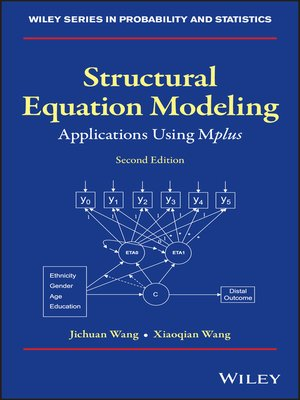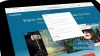# Structural Equation Modeling

### Applications Using Mplus ·Wiley in Probability and Statistics

#### byJichuan WangAuthor · Xiaoqian WangAuthor

ebook

With an OverDrive account, you can save your favorite libraries for at-a-glance information about availability. Find out more about OverDrive accounts.

### Subjects

Presents a useful guide for applications of SEM whilst systematically demonstrating various SEM models using Mplus

Focusing on the conceptual and practical aspects of Structural Equation Modeling (SEM), this book demonstrates basic concepts and examples of various SEM models, along with updates on many advanced methods, including confirmatory factor analysis (CFA) with categorical items, bifactor model, Bayesian CFA model, item response theory (IRT) model, graded response model (GRM), multiple imputation (MI) of missing values, plausible values of latent variables, moderated mediation model, Bayesian SEM, latent growth modeling (LGM) with individually varying times of observations, dynamic structural equation modeling (DSEM), residual dynamic structural equation modeling (RDSEM), testing measurement invariance of instrument with categorical variables, longitudinal latent class analysis (LLCA), latent transition analysis (LTA), growth mixture modeling (GMM) with covariates and distal outcome, manual implementation of the BCH method and the three-step method for mixture modeling, Monte Carlo simulation power analysis for various SEM models, and estimate sample size for latent class analysis (LCA) model.

The statistical modeling program Mplus Version 8.2 is featured with all models updated. It provides researchers with a flexible tool that allows them to analyze data with an easy-to-use interface and graphical displays of data and analysis results.

Intended as both a teaching resource and a reference guide, and written in non-mathematical terms, Structural Equation Modeling: Applications Using Mplus, 2nd edition provides step-by-step instructions of model specification, estimation, evaluation, and modification. Chapters cover: Confirmatory Factor Analysis (CFA); Structural Equation Models (SEM); SEM for Longitudinal Data; Multi-Group Models; Mixture Models; and Power Analysis and Sample Size Estimate for SEM.

• Presents a useful reference guide for applications of SEM while systematically demonstrating various advanced SEM models
• Discusses and demonstrates various SEM models using both cross-sectional and longitudinal data with both continuous and categorical outcomes
• Provides step-by-step instructions of model specification and estimation, as well as detailed interpretation of Mplus results using real data sets
• Introduces different methods for sample size estimate and statistical power analysis for SEM
• Structural Equation Modeling is an excellent book for researchers and graduate students of SEM who want to understand the theory and learn how to build their own SEM models using Mplus

### Publication Details

Publisher:
Wiley
Edition:
2
Publication Date:
2019
Series:
Wiley in Probability and Statistics

### Format